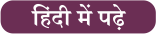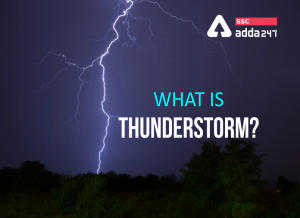Latest SSC jobs   »   Force: Definition, Unit, Formula, Effects And...

# Force: Definition, Unit, Formula, Effects And Types

## What is Force?

A force is an interaction of the object with mass that causes the object to change its velocity. It can be defined as the push or pull on a particular object. A force is a vector quantity which means it has both magnitude and direction. When objects interact with one another, it is likely to cause a push or pull resulting in the Force. For example, when you try to push a ball, it causes a force on the ball that changes its state of motion. The external force applied has the capability to change the position of a particular object. The direction in which the force is applied is known as the direction of Force. In this post, we will discuss everything related to Force including its Formula and Types. Check out the article below to know about Force.

## Unit of Force

The SI unit of Force is Newton(N). However, Force can also be defined in various other units as given in the table below.

 Common symbols: F→, F SI unit Newton In SI base units: kg·m/s2 Other units dyne, poundal, pound-force, kip, kilopond Derivations from other quantities F = m a Dimension LMT-2

Periodic Table: Groups, Properties And Laws

## Effects of Force

Whenever a Force is applied on any object, it tends to change its shape, size, speed or direction. It changes the motion of an object. Motion is the movement of a body. Here are some of the effects that force has on its objects:

1. It changes the direction of an object.
2. It can make a body at rest to move.
3. It can change the speed of an object by either increasing or decreasing it.
4. It can stop a moving object.
5. It can change the shape or size of an object.

## Formula of Force

Force can be explained by the product of mass (m) and acceleration (a). The equation for the formula of Force can be expressed in the form as given below:

### F = ma

Where,

• m = mass
• a = acceleration

It is formulated in Newton (N) or Kgm/s2.

Acceleration “a” is given by, a = v/t

Where

• v = velocity
• t = time taken

So Force can be defined as F = mv/t

Inertia formula is termed as p = mv which can also be explained as Momentum.

## Types of Force

There are various types of Force that act on an object. Here are some of Force applied on an object generally:

1. Muscular Force
2. Mechanical Force
3. Frictional Force
4. Gravitational Force
5. Electrostatic Force
6. Magnetic Force

Let’s take a look at the Types of Force in detail:

1. Muscular Force

It can be simply defined as the force that we apply on a daily basis by the work we do such as lifting, breathing, exercising etc. It acts after coming in contact with the object. This force results due to the action of our muscles. When we use our muscles to do the daily chores, muscular force is exerted on the object.

2. Mechanical Force

The mechanical force occurs when there is direct contact between two objects where one object is applying the force while the other one is in a state of rest or in the state of motion. Someone pushing the door is an example of Mechanical force.

3. Frictional Force

Frictional Force is the opposing force created between two surfaces whose main purpose is to create resistance to the object either moving in the same direction or opposite direction.  When we put brakes while riding a cycle act as a frictional force.

4. Gravitational Force

Gravitational Force is the force of attraction that attracts two objects with masses. Earth’s gravitational force pulls us to the ground. It always tries to pull the masses towards each other and never tries to push them apart. It can be defined as Newton’s Universal Law of Gravitation.

5. Electrostatic Force

Electrostatic Force is also defined as the Coulomb force or Coulomb interaction. It is the force between two electrically charged objects. According to this force, like charges repel while unlike charges attract each other. Lightning is an example of the Electrostatic Force.

6. Magnetic Force

Magnetic Force is the force that arises between electrically charged particles because of their motion. Magnetic force can be seen between the poles of 2 magnets. It is attractive or repulsive in nature depending upon the orientation of the object. It can be said to be a consequence of Electrostatic Forces.Preparing for SSC Exams in 2021? Register now to get free study material

#### Congratulations!

General Awareness & Science Capsule PDF

Incorrect details? Fill the form again here

General Awareness & Science Capsule PDF

Thank You, Your details have been submitted we will get back to you.
•UPSSSC PET 2022 Notification Out, Online...
•SSC MTS Cut Off 2022, Tier I Cut off Mar...
•SSC MTS Study Plan : Start Your Practice...
•What is Thunderstorm? Causes, Types, And...
•International Yoga Day 2022, 21st June T...
•World Music Day 2022, 21st June, Theme, ...